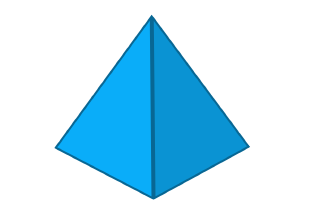# Polyhedron - Definition with Examples

The Complete K-5 Math Learning Program Built for Your Child

• 30 Million Kids

Loved by kids and parent worldwide

• 50,000 Schools

Trusted by teachers across schools

• Comprehensive Curriculum

Aligned to Common Core

Polyhedron

A polyhedron is a 3-dimensional solid made by joining together polygons.

The word 'polyhedron' comes from two Greek words,  poly meaning many, and hedron referring to surface.

The polyhedrons are defined by the number of faces it has.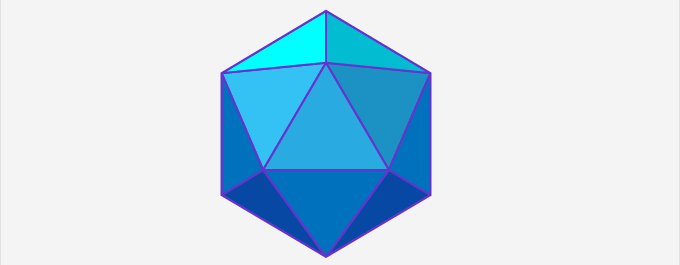An example of a polyhedron

Parts of a polyhedron

Every polyhedron has three parts:

• Face: the flat surfaces that make up a polyhedron are called its faces. These faces are regular polygons.
• Edge: the regions where the two flat surfaces meet to form a line segment are known as the edges.
• Vertex: It is the point of intersection of the edges of the polyhedron. A vertex is also known as the corner of a polyhedron. The plural of vertex is called vertices.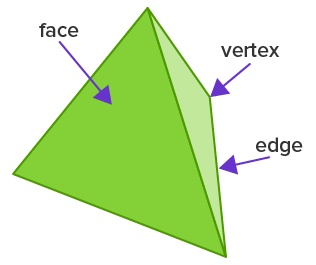The names of the polyhedrons are derived from the number of faces they have:

 Name No. of faces Tetrahedron A polyhedron with 4 faces. Pentahedron A polyhedron with 5 faces. Hexahedron A polyhedron with 6 faces. Heptahedron A polyhedron with 7 faces. Octahedron A polyhedron with 8 faces. Nonahedron A polyhedron with 9 faces. Decahedron A polyhedron with 10 faces

Types of polyhedron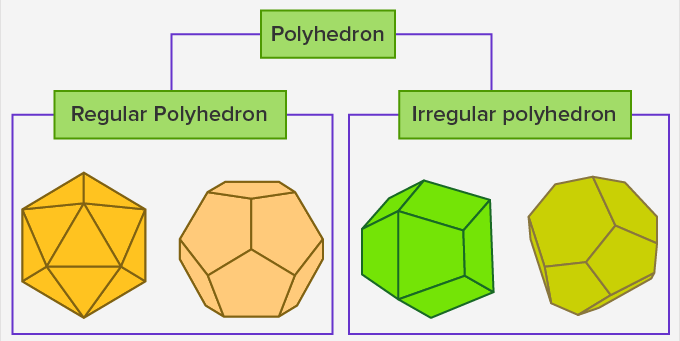Regular Polyhedron

A regular polyhedron is made up of regular polygons. Such solids are also known as ‘platonic solids’

Irregular polyhedron

An irregular polyhedron is formed by polygons of different shapes where all the components are not the same. This means that all the sides of an irregular polyhedron are not equal.

Some of the regular polygons are described in the table below:

Polyhedrons in everyday life

 Examples Images Houses/Buildings: Most of the houses around us are polyhedrons as they are made of flat surfaces.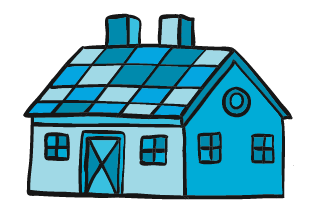A prism: A  prism is made of three rectangles joined together with triangular bases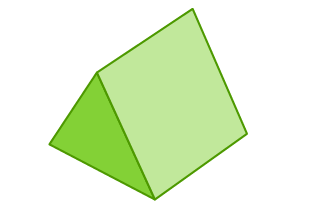A football or a soccer ball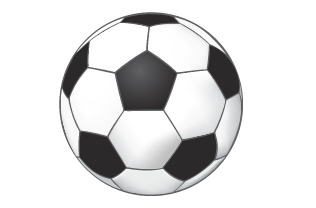Pyramids: A polyhedron with four sides is a tetrahedron and is also called a pyramid.